﻿ Thermal Expansion in Zinc Oxide Nanomaterials

### Thermal Expansion in Zinc Oxide Nanomaterials

Mahipal Singh, Madan SinghOPEN ACCESSPEER-REVIEWED

## Thermal Expansion in Zinc Oxide Nanomaterials

Mahipal Singh1,, Madan Singh2

1Department of Physics, Government Post Graduate College, Bageshwar, Uttarakhand, India

2Department of Physics and Electronics, National University of Lesotho, Roma, Lesotho, Southern Africa

### Abstract

Thermal expansion of rock salt and wurtize phases of Zinc Oxide nanomaterials has been studied using various relationships between volume thermal expansivity and temperature. The numerical values of volume thermal expansion coefficient for rs-ZnO and w-ZnOnanomaterials have been calculated in low and high temperature ranges. It is observed that in low temperature range i.e. upto room temperature, volume thermal expansion coefficient increases with slow rate with temperature for both the phases of Zinc Oxide while in high temperature range, thermal expansion coefficient increases with high rate with increase in temperature in the case of rs-ZnO and with slow rate in the case of w-ZnO. The available experimental and theoretical research support these theoretical predictions that demonstrates the validity of the work.

### At a glance: Figures

1
Prev Next

• Singh, Mahipal, and Madan Singh. "Thermal Expansion in Zinc Oxide Nanomaterials." Nanoscience and Nanotechnology Research 1.2 (2013): 27-29.
• Singh, M. , & Singh, M. (2013). Thermal Expansion in Zinc Oxide Nanomaterials. Nanoscience and Nanotechnology Research, 1(2), 27-29.
• Singh, Mahipal, and Madan Singh. "Thermal Expansion in Zinc Oxide Nanomaterials." Nanoscience and Nanotechnology Research 1, no. 2 (2013): 27-29.

 Import into BibTeX Import into EndNote Import into RefMan Import into RefWorks

### 1. Introduction

Over the past decade, nanomaterials have been the subject of enormous interest. Due to their extremely small size, these materials have the potential for wide-ranging industrial, biomedical, electronic etc. applications. Recently, Zinc Oxide (ZnO) has attracted much attention within the scientific community as a ‘future material’. This is however, somewhat of a misnomer, as ZnO has been widely studied since 1935 with much of our current industry and day-to-day lives critically reliant upon this compound .

Zinc Oxide (ZnO) belongs to the family of wide-bandgap semiconductors with strong ionic character of chemical bonds. At ambient conditions ZnO has a wurtize structure (P63mc) that transforms into a rock-salt one (Fm3m) at high pressures . Material scientists and technologists have started to study the various properties of ZnOnanomaterials under different conditions. Recently, it is observed that volume thermal expansion coefficient of ZnO increases with high rate with increase in temperature .

The thermal expansion of rock-salt ZnO, metastable high-pressure phase, has been experimentally studied in 10-300 K temperature range at ambient pressure using synchrotron x-ray powder diffraction. The volume thermal expansion coefficients is found to increase from almost zero value in 10-80 K range to 4.77 x 10-5 K-1 at 298 K and remains virtually constant at higher temperatures.

A lot of work is required in this direction because of numerous applications of ZnO. Therefore, it is planned to study the thermal expansion in ZnOnanomaterials under different temperature range.

### 2. Method of Analysis

The relationship between volume thermal expansivity and temperature is given as [4, 5]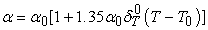(1)

Where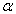is volume thermal expansivity at temperature T,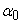volume thermal expansivity at room temperature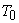and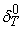Anderson-Gruneisen parameter at room temperature.

It has been noted that under the effect of temperature, the product of thermal expansion coefficient coefficient () and isothermal bulk modulus(K) for nanomaterials remains constant  i.e.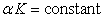(2)

Differentiating Equation(2) with respect to T, we get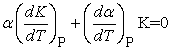(3)

The Anderson-Gruneisen parameter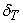is defined as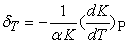(4)

From Equations (3) and (4), we have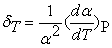(5)

Mathematical definition of volume thermal expansivity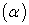is given as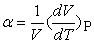(6)

Or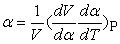Or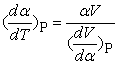(7)

From Equation (5) and (7), we have-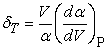(8)

The temperature dependence ofis represented as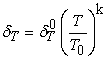(9)

Here ‘k’ is new dimensionless thermoelastic parameter whose value is calculated by the slope of the graph plotted between log() and log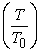.

Taking log on both sides of Equation(9), we get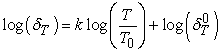(10)

Obviously, the graph plotted between log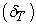and log(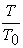) is a straight line whose slope gives the value of ‘k’. Therefore,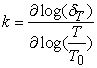(11)

From Equation (5) and (9), we have-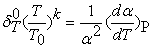(12)

Integrating Equation (12), we get-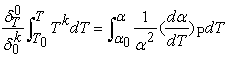(13)

On solving the integration and rearranging the terms, we get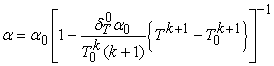(14)

Using above expressions (1) and (14), the value of volume thermal expansivity () for rs-ZnO and w-ZnOnanomaterialsis evaluated directly as a function of temperature (T).

### 3. Results and Discussion

Using Equation (1) and (14), the values of volume thermal expansion coefficient for both the phases of ZnOnanomaterials have been calculated in low as well as high temperature range i.e. in the temperature range from 10 K to 2100 K. The input parameters used in the computation have been given in Table 1.

#### Table 1. Input parameters used in computation [5,10,11,12]Download asPowerPoint Slide

Veiw figureView current table in a new window

The theoretical results obtained are reported in Figure (1). Figure 1(a) represents the variation of volume thermal expansivity () with temperature (T) for rs-ZnO nanomaterial according to Equation (1) while Figure 1(b) represents this variation forrs-ZnO nanomaterial as per Equation (14). It is obvious that the results obtained from Equation (1) are in good agreement with the results obtained from Equation (14). Similarly, Figures 1(c) and 1(d) report the results obtained from Equation (1) and (14) respectively for w-ZnO nanomaterial. It is also obvious that the theoretical results obtained from Equations (1) and (14) are in good agreement with each other. It is observed that in low temperature range i.e. upto room temperature, volume thermal expansion coefficient increases with slow rate with temperature for both the phases of Zinc Oxide nanomaterialswhile in high temperature range, thermal expansion coefficient increases with high rate with increase in temperature in the case of rs-ZnOnanomaterial and with slow rate in the case of w-ZnO nanomaterial. These theoretical results on volume thermal expansivity of rs-ZnO and w-ZnOnanomaterials are in good agreement with the existing experimental results [2, 3] which demonstrates the validity of the work. Our theoretical results may stimulate the experimental scientists for the measurement of variation of volume thermal expansivity with temperature for different nanomaterials in the future.

The present work may be extended to evaluate other mechanical and thermodynamical properties of ZnOnanomaterials and other nanomaterials and may be used for comparative study of nanomaterials.

### 4. Conclusion

In the present work, the behavior of volume thermal expansivity as a function of temperature for both phases of ZnOnanomaterials in the low as well as high temperature range has been studied using different Equations. It is observed that in low temperature range i.e. upto room temperature, volume thermal expansion coefficient increases with slow rate with temperature for both the phases of Zinc Oxidenanomaterials while in high temperature range, thermal expansion coefficient increases with high rate with increase in temperature in the case of rs-ZnOnanomaterial and with slow rate in the case of w-ZnO nanomaterial. These theoretical results on volume thermal expansivity are in good agreement with the existing experimental results which demonstrates the validity of the work.

Figure 1. Variation of volume thermal expansivity ( α ) as a function of temperature (T) for rs-ZnO and w-ZnO nanomaterials

### References

  C.W. Bunn, “A Comparative Review of ZnO Materials and Devices”, Proc. Phys.Soc., London, 47, 1935, 835.In article CrossRef  V.L. Solozhenko, O.O. Kurakevich, P.S. Sokolov and A.N. Baranov, “Kinetics of the Wurtzite-to-Rock-Salt Phase Transition in ZnO at High Pressure”, J. Phys. Chem.A, 115, 2011, 4354.In article CrossRef PubMed  PetrS.Sokolov, Andrey N. Baranov, Anthony M.T. Bell and Vladimir L. Solozhenko, “Low-Temperature Thermal Expansion of Rock-Salt ZnO “, Proc.Jpn.Acad. B, 75, 1999, 1.In article  ChuanhuiNie, Shangyong Huang and Wei Huang, “Temperature Dependence of Anderson-Gruneisen Parameter for NaCl”, Appl. Phys. Research, 2(1), 2010, 144.In article  Mahipal Singh, “Analysis of Temperature Dependence of Anderson-Gruneisen Parameter for ZnONanorods”, International J. Phys. and Research, 3(4), 2013, 55.In article  O.L. Anderson and K. Zou, “Formulation of the thermodynamic Function for Mantle Minerals: MgO as an Example”, Phys. Chem. Miner. 16, 1989, 642.In article CrossRef  O.L. Anderson, “Equation of State for Geo Physics and Ceramic Science” Oxford University Press, New York, 1995.In article  S.K. Srivastava, PallaviSinha and Monika Panwar, “Thermal Expansivity and Isothermal Bulk Modulus of Ionic Materials at High Temperatures”; Indian J. Pure and Appl. Phys. 47, 2009, 175.In article  Jeewan Chandra, DeepikaKandpal, B.R.K. Gupta, “Study of Thermo-Elastic Properties of NanomaterialsUnder High Temperature”, Physica B, 404, 2009, 1087.In article CrossRef  A. Seko, F. Oba, A. Kuwabara and I. Tanaka, “Pressure-Induced Phase Transition in ZnO and ZnO-MgO Pseudo-Binary System: A First Principles Lattice Dynamics Study”, Phys. Rev. B, 72, 2005, 024107.In article CrossRef  D. Taylor, “Negative Thermal Expansion Materials”, Br. Ceram. Trans., 83, 1984, 5.In article  Mahipal Singh, “Study of Temperature Dependence of Isothermal Bulk Modulus of ZnONanomaterials”, International J. Adv. Research in Sci. and Engg., 2(10), 2013, 109.In article